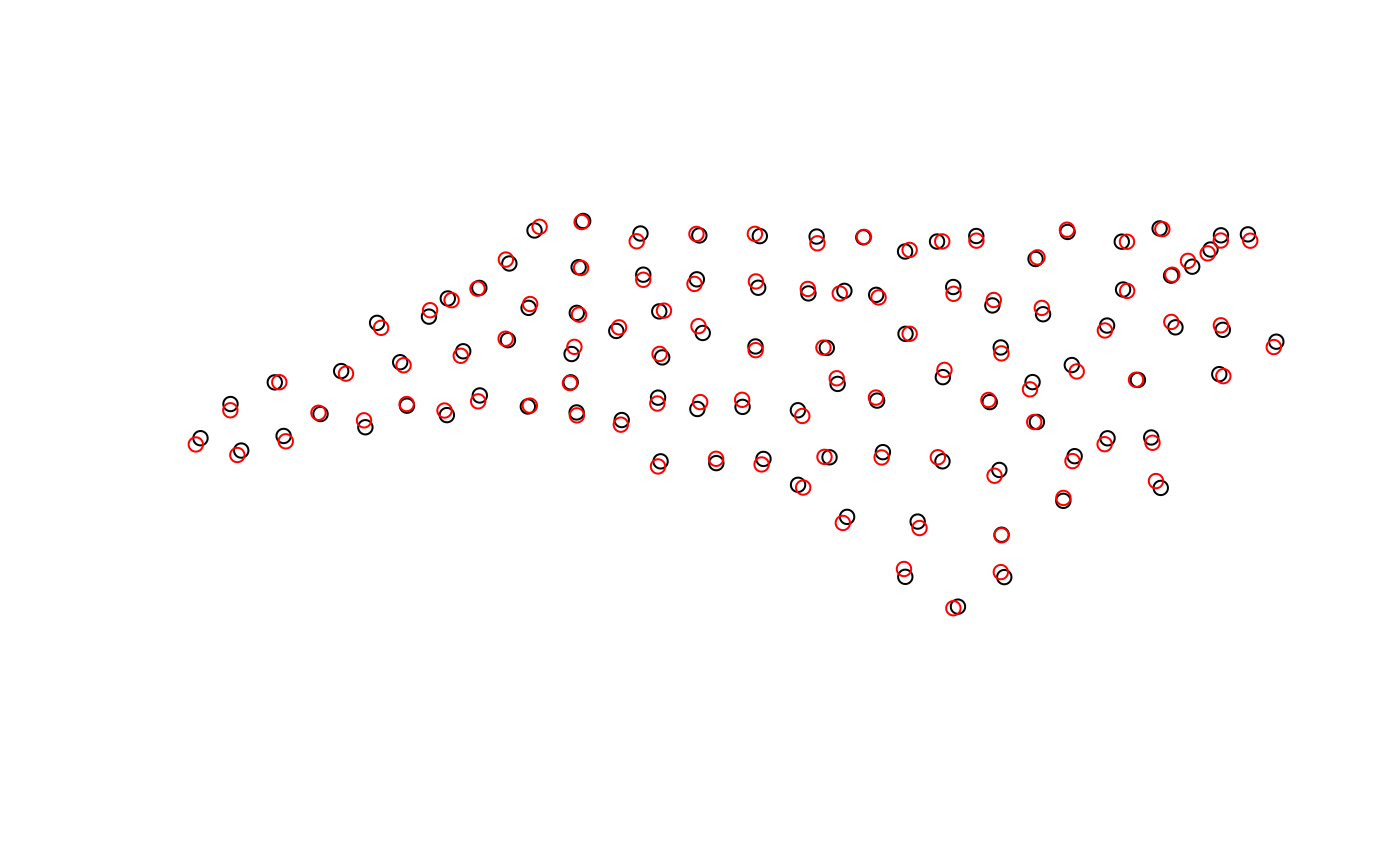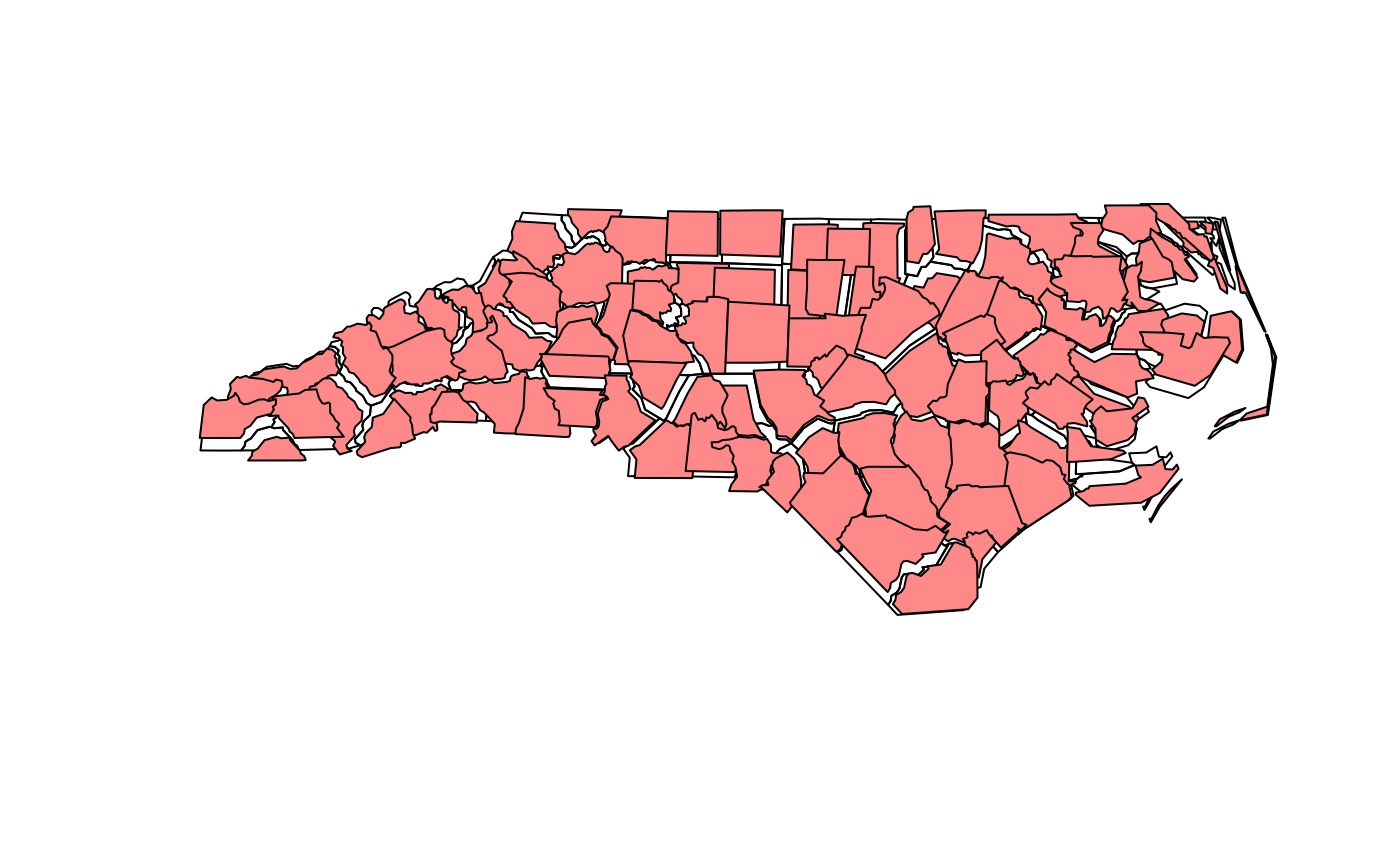jitter geometries

st_jitter(x, amount, factor = 0.002)

## Arguments

x

object of class sf or sfc

amount

numeric; amount of jittering applied; if missing, the amount is set to factor * the bounding box diagonal; units of coordinates.

factor

numeric; fractional amount of jittering to be applied

## Details

jitters coordinates with an amount such that runif(1, -amount, amount) is added to the coordinates. x- and y-coordinates are jittered independently but all coordinates of a single geometry are jittered with the same amount, meaning that the geometry shape does not change. For longlat data, a latitude correction is made such that jittering in East and North directions are identical in distance in the center of the bounding box of x.

## Examples

nc = read_sf(system.file("gpkg/nc.gpkg", package="sf"))
pts = st_centroid(st_geometry(nc))
plot(pts)
plot(st_jitter(pts, .05), add = TRUE, col = 'red')plot(st_geometry(nc))
plot(st_jitter(st_geometry(nc), factor = .01), add = TRUE, col = '#ff8888')